# Numerical Software

### Mathematics Software – Free alternatives

Numerical software is specialized code used to analyze, model, or compute numeric, symbolic, or geometric information. Many numerical suites are computer algebra systems that use symbolic algebra. They are designed to solve classical equations and tasks in close-to-common notation. This is a short list of helpful options...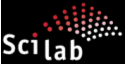Scilab - www.scilab.org
Scilab is an open source software with a GPL compatible licence. The Scilab Consortium is involved in the Open Source world in a number of ways. Libraries are free with accepted and recognized licenses by the Free, Libre, Open Source Software (FLOSS) community.Scicoslab – www.scicoslab.org
ScicosLab is the new name of ScilabGtk. This change of name has been taken in order to avoid confusion with Scilab, which is no longer developed at INRIA. ScicosLab is used in particular for disributing new software developments from research activities of the Metalau team at INRIA and ENPC, such as Scicos and the Maxplus toolbox.Octave - gnu.org/software/octave GNU Octave is a high-level interpreted language, intended for numerical computations. It provides capabilities for the numerical solution of linear and nonlinear problems, and for performing other numerical experiments. It also provides graphics capabilities for data visualization and manipulation. The Octave language is quite similar to Matlab so that most programs are easily portable.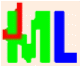JMathLib - www.jmathlib.de
JMathLib is a clone of Matlab, but written entirely in java.  A library of functions designed to be used in evaluating complex expressions and display the results graphically. It’ll be used either interactively through a terminal like window or to interpret script files. It is intended to be a java version of programs such as Matlab, Scilab, Octave, and FreeMat.Maxima - maxima.sourceforge.net
Maxima is a software for the manipulation of symbolic and numerical expressions, including differentiation, integration, Laplace transforms, differential equations, systems of linear equations, polynomials, lists, vectors, matrices, and tensors. Maxima produces high precision numeric results by using exact fractions, arbitrary precision integers, and variable precision floating point numbers. It can plot functions and data in 2D and 3D. Maxima is a descendant of Macsyma, the computer algebra system developed at the MIT.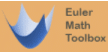Euler - eumat.sourceforge.net
The Euler Mathematical Toolbox is a powerful, mature software for numerical and symbolic computations. Euler is similar to Matlab, but has an own style, and a slightly different syntax. Symbolic mathematics is supported in Euler using the open algebra system Maxima.FreeMat - freemat.sourceforge.net
FreeMat is an environment for rapid engineering and scientific prototyping and data processing. Similar to commercial systems such as Matlab and IDL, but it’s open source. It’s available under the GPL license.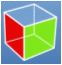GTK+ - www.gtk.org
GTK+ is a highly usable toolkit for creating graphical user interfaces. It has an easy to use API and it’s written in C, but has bindings to many other popular programming languages such as C++, Python and C# among others. GTK+ has been involved in many projects and some big platforms.The Algae Programming Language - algae.sourceforge.net
A programming language for numerical analysis. Algae was developed because of the need of a fast and versatile tool, capable of handling large systems. Algae has been applied to interesting problems in aerospace and related fields for more than a decade.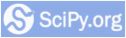Python and Scientific Computing - www.scipy.org
NumPy and SciPy are two of many open-source packages for scientific computing that use the Python programming language.

From 'Numerical Software' to home

From 'Numerical Software' to Computer Programming Basics

 Top Open Source Books